Processing ......FreeComputerBooks.com Links to Free Computer, Mathematics, Technical Books all over the World

From Algorithms to Z-Scores: Probabilistic and Statistical Modeling in Computer Science
🌠 Top Free Mathematics Books - 100% Free or Open Source!
• Title: From Algorithms to Z-Scores: Probabilistic and Statistical Modeling in Computer Science
• Author(s) Norm Matloff
• Publisher: Orange Grove Texts Plus (September 24, 2009); eBook (Creative Commons Edition, 2013)
• License(s): CC BY-ND 3.0 US
• Paperback: 274 pages
• eBook: PDF (543 pages)
• Language: English
• ISBN-10: 1616100362
• ISBN-13: 978-1616100360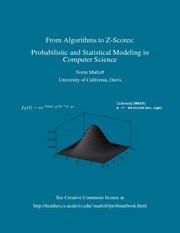Book Description

This is a textbook for a course in mathematical probability and statistics for computer science students. Computer science examples are used throughout, in areas such as: computer networks; data and text mining; computer security; remote sensing; computer performance evaluation; software engineering; data management; etc.

• Norm Matloff is an American professor of computer science at the University of California, Davis. He was formerly a statistics professor at that university, and thus approaches the subject matter here as both a statistician and computer scientist.
Reviews, Ratings, and Recommendations: Related Books and Categories: Read and Download Links:Similar Books:
•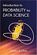Introduction to Probability for Data Science (Stanley Chan)

This book is an introductory textbook in undergraduate probability in the context of data science to emphasize the inseparability between data (computing) and probability (theory) in our time, with examples in both MATLAB and Python.

•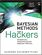Bayesian Methods for Hackers: Probabilistic Programming

This book illuminates Bayesian inference through probabilistic programming with the powerful PyMC language and the closely related Python tools NumPy, SciPy, Matplotlib, through practical examples and computation - no advanced mathematics required.

•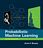Probabilistic Machine Learning: An Introduction (Kevin Murphy)

This book is a comprehensive introduction to machine learning that uses probabilistic models and inference as a unifying approach. It is written in an informal, accessible style, complete with pseudo-code for the most important algorithms.

•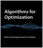Algorithms for Optimization (Mykel J. Kochenderfer, et al.)

A comprehensive introduction to optimization with a focus on practical algorithms for the design of engineering systems. Readers will learn about computational approaches for a range of challenges.

•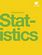Introductory Statistics (OpenStax)

This book is geared toward students majoring in fields other than math or engineering. This text assumes students have been exposed to intermediate algebra, and it focuses on the applications of statistical knowledge rather than the theory behind it.

•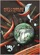Mostly Harmless Statistics (Rachel L. Webb)

This text is for an introductory level probability and statistics course with an intermediate algebra prerequisite. The focus of the text follows the American Statistical Association's Guidelines for Assessment and Instruction in Statistics Education (GAISE).

•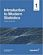Introduction to Modern Statistics (Mine Çetinkaya-Rundel, et al.)

This book puts a heavy emphasis on exploratory data analysis and provides a thorough discussion of simulation-based inference using randomization and bootstrapping, followed by a presentation of the related Central Limit Theorem based approaches.

•Foundations in Statistical Reasoning (Pete Kaslik)

This book is designed for students taking an introductory statistics class. The emphasis throughout the entire book is on how to make decisions with only partial evidence. It focuses on the thought process.

•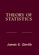Theory of Statistics (James E. Gentle)

This book is directed toward students for whom mathematical statistics is or will become an important part of their lives. It covers classical likelihood, Bayesian, and permutation inference; an introduction to basic asymptotic distribution theory; and modern topics.

•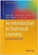Introduction to Statistical Learning: with Applications in Python

This book covers the same materials as Introduction to Statistical Learning: with Applications in R (ISLR) but with labs implemented in Python. These labs will be useful both for Python novices, as well as experienced users.

•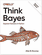O'Reilly® Think Bayes: Bayesian Statistics in Python

If you know how to program, you're ready to tackle Bayesian statistics. With this book, you'll learn how to solve statistical problems with Python code instead of mathematical formulas, using discrete probability distributions rather than continuous mathematics.

Book Categories
 :All CategoriesTop Free BooksRecent BooksMiscellaneous BooksComputer EngineeringComputer LanguagesComputer ScienceData Science/DatabasesJava and Java EE (J2EE)Linux and UnixMathematicsMicrosoft and .NETMobile ComputingNetworking and CommunicationsSoftware EngineeringSpecial TopicsWeb Programming
Other Categories